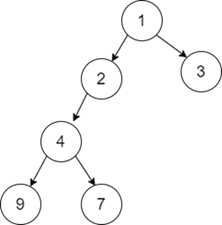# Find the sum of left leaf nodes of a given Binary Tree in C++

C++Server Side ProgrammingProgramming

Let us suppose we have a Binary Tree having a root node and its left child and right child. The task is to find the total sum of leaf nodes of the tree which are left to its parent node.

For Example

Input-1:Output:

15

Explanation: In the given input Binary Tree, the sum of all the left leaf nodes is 9+4+2 = 15. So, the output is 15.

## Approach to Solve this Problem

We have a Binary Tree and the task is to find the sum of all the leaf nodes which are left to its parent.

The recursive approach to solve this problem is to check if the left node of the root node is empty or not. If it is empty, then calculate the sum for its left node and find the recursive sum for the right node. Thus, for every node, we will check recursively and find its sum.

• Take a Binary Tree having root nodes and its left child as well as right child as the input.
• An integer function leftLeafSum(treenode*root) takes the root node as the input and returns the sum of all the leaf nodes which are left to its parent.
• If the root node is empty or NULL, then return 'zero' otherwise check for the left node of the root node.
• If the left node of the root node is having no children, then recursively check for the right node.
• Return the sum recursively for the left child and the right child.

## Example

Live Demo

#include<bits/stdc++.h>
using namespace std;
struct treenode {
int data;
treenode * left;
treenode * right;
};
struct treenode * createNode(int d) {
struct treenode * root = new treenode;
root -> data = d;
root -> left = NULL;
root -> right = NULL;
return root;
}
int leftLeafSum(treenode * root) {
if (root == NULL) {
return 0;
}
if (root -> left and!root -> left -> left and!root -> left -> right) {
return root -> left -> data + leftLeafSum(root -> right);
}
return leftLeafSum(root -> left) + leftLeafSum(root -> right);
}
int main() {
struct treenode * root = NULL;
root = createNode(4);
root -> left = createNode(2);
root -> right = createNode(2);
root -> left -> right = createNode(7);
root -> left -> left = createNode(5);
root -> right -> left = createNode(5);
root -> right -> right = createNode(7);
int sum = leftLeafSum(root);
cout << sum << endl;
return 0;
}

Running the above code will generate the output as,

## Output

10

Explanation: The leaf nodes in the left nodes are 5 and 5 which are left to their parent, thus the sum of all the leaf node is = 10.